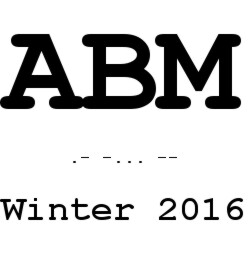###### Links und Funktionen

• Startseite
• Arbeitstagung Bern–MĂĽnchen
Winter 2016

# Arbeitstagung Bern–MĂĽnchen Winter 2016

December 8–9, 2016
Conference room B 349
Mathematisches Institut LMU
Theresienstr. 39

Logic and Theory Group
UniversitĂ¤t Bern
Arbeitsgruppe Mathematische Logik
LMU MĂĽnchen## Programme

### Wednesday 07.12

 19:30 Welcome dinner at Alter Simpl TĂĽrkenstraĂźe 57

### Thursday 08.12

 09:10 Opening 09:15â€“10:00 M. Marti â€” Intuitionistic Justification Logics: Atomic Models and Realization without Variables We consider an intuitionistic justification logic and some problems regarding the transformation of models to so-called atomic models and realization without variables. 10:15â€“11:00 S. Steila â€” On $\Sigma_1$-fixed point statements in Kripke–Platek In Kripke Platek Set Theory (KP), every monotone, bounded $\Sigma_1$-formula has a $\Sigma_1$-definable least fixed point. Hence $\Sigma_1$-separation is strong enough to prove that the least fixed point is actually a set. To the aim of understanding the relation between these two principles, we perform an analysis of some distinct fixed-points-statements over KP. (joint work with Gerhard JĂ¤ger) 11:15â€“12:00 M. BĂ¤rtschi â€” From transfinite dependent choice to fixpoints of monotone operators We introduce two formal systems based on $\mathsf{ACA_0}$, namely the system $\mathsf{{weak-} \Sigma_1^1 {-} TDC}$, standing for weak $\Sigma_1^1$ transfinite dependent choice, and the system $\mathsf{M \Delta_1^1 {-} FP_0}$, featuring a fixed point scheme for monotone $\Delta_1^1$ operators. We will show that working in $\mathsf{{weak-} \Sigma_1^1 {-} TDC}$ we can derive the fixed point scheme of $\mathsf{M \Delta_1^1 {-} FP_0}$. Moreover, relations to other formal systems will be discussed briefly. (joint work with Gerhard JĂ¤ger)
 12:15â€“14:00 Lunch break
 14:15â€“15:00 I. Petrakis â€” An Inequality Type in Intensional Type Theory Motivated by the importance of apartness relations in constructive mathematics we introduce an inequality type into Martin-LĂ¶f's Intensional Type Theory. The induction axiom of this type corresponds to the induction axiom of the equality type. We present some first results on the inequality type and we discuss its interpretation within Homotopy Type Theory. 15:15â€“16:00 L. Jaun â€” Constructions for Identity-Elimination in Explicit Mathematics I will present the identity-elimination rule from Martin-LĂ¶f type theory in the context of explicit mathematics; the system BON extended with classes. I will describe why it is uninteresting to consider the elimination axiom if it applies to all explicit mathematics-classes and then give some constructions which can serve as a starting point for the investigation of identities in explicit mathematics.
 16:10â€“16:30 Coffee break
 16:30â€“17:15 D. Rinaldi â€” Extension by Conservation. Sikorski's Theorem We give constructive meaning to the classical assertion that every complete and atomic Boolean algebra is an injective object in the category of distributive lattices. To this end, we employ Scott's notion of entailment relation, in which context we describe Sikorski's extensions theorem for finite Boolean algebras and turn it into a syntactical conservation result. (joint work with Daniel Wessel)
 19:30 Dinner at Max Emanuel AdalbertstraĂźe 33

### Friday 09.12

 09:15â€“10:00 B. Pientka â€” Mechanizing Meta-Theory in Beluga Mechanizing formal systems, given via axioms and inference rules, together with proofs about them plays an important role in establishing trust in formal developments. In this talk, I will survey the proof environment Beluga. To specify formal systems and represent derivations within them, Beluga provides a sophisticated infrastructure based on the logical framework LF; to reason about formal systems, Beluga provides a dependently typed functional language for implementing inductive proofs about derivation trees as recursive functions following the Curry-Howard isomorphism. Key to this approach is the ability to model derivation trees that depend on a context of assumptions using a generalization of the logical framework LF, i.e. contextual LF which supports first-class contexts and simultaneous substitutions. Our experience has demonstrated that Beluga enables direct and compact mechanizations of the meta-theory of formal systems, in particular programming languages and logics. To demonstrate Beluga's strength in this talk, we develop a weak normalization proof using logical relations. 10:15â€“11:00 K. Miyamoto â€” Implementing a logic for proof complexity analysis Hilbert's epsilon calculus is a first-order logic enriched with the epsilon operator $\varepsilon$. The epsilon operator allows to form terms $\varepsilon_x A(x)$ whose property is characterized by the critical axiom $A(t) \to A(\varepsilon_x A(x))$ where $t$ is an arbitrary term. Through analyzing the epsilon elimination process used in the proofs of the conservativity results of Hilbert's epsilon calculus, namely, the first and second epsilon theorems, Moser and Zach obtained the bound of the size of Herbrand disjunctions. We talk about their results and its computer implementation which is my work in progress. 11:15â€“12:00 J. Berger â€” Brouwer's fan theorem and convexity Let $X$ be the set of finite binary sequences. We investigate the relationship between decidable subsets of $X$ and uniformly continuous functions on the unit interval. (joint work with Gregor Svindland) 12:15 Closing

## Participants

 Michael Bärtschi, Bern Josef Berger, LMU Wilfried Buchholz, LMU Andreas Franz, LMU Gerhard JĂ¤ger, Bern Lukas Jaun, Bern Basil Karádais, LMU Michel Marti, Bern Kenji Miyamoto, Innsbruck Iosif Petrakis, LMU Brigitte Pientka, McGill/LMU Davide Rinaldi, Verona Timotej Rosebrock, Bern Kentaro Sato, Bern Nenad SaviÄ‡, Bern Peter Schuster, Verona Helmut Schwichtenberg, LMU Silvia Steila, Bern Daniel Wessel, Trento Chuangjie Xu, LMU Wolfgang Zuber, LMU

• M. Bärtschi
• B. Karádais